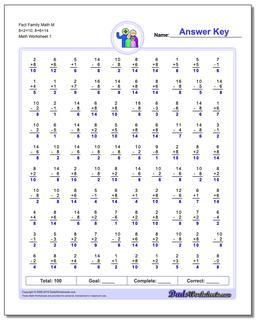# Math Worksheets: Fact Family Math: Fact Family Math: Fact Family Math M 8+2=10, 8+6=14## Fact Family Math M 8+2=10, 8+6=14

PropertyValue
DescriptionFact Family Math M 8+2=10, 8+6=14: These math worksheets have 100 addition and subtraction fact family problems and make for a challenging two minute test.
Resource TypeWorksheet